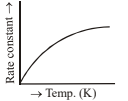# NEET Chemistry Chemical Kinetics Questions Solved

If for a first order reaction, rate constant varies with temperature according to the graph given below. At $27°C$$1.5×{10}^{-4}$ percent of the reactant molecules are able to cross-over the potential barrier. At $52°C$, the slope of this graph is equal to , calculate the value of rate constant at $52°C,$ assuming that activation energy does not change in this temperature range.(A)

(B)

(C)

(D)

Concept Videos :-

#8 | Problems on 1st Order Reaction (Type 1)
#9 | Problems on 1st Order Reaction (Type 2)
#10 | Problems on 1st Order Reaction (Type 3)
#12 | Problems on 1st Order Reaction (Type 5)

Concept Questions :-

First Order Reaction Kinetics